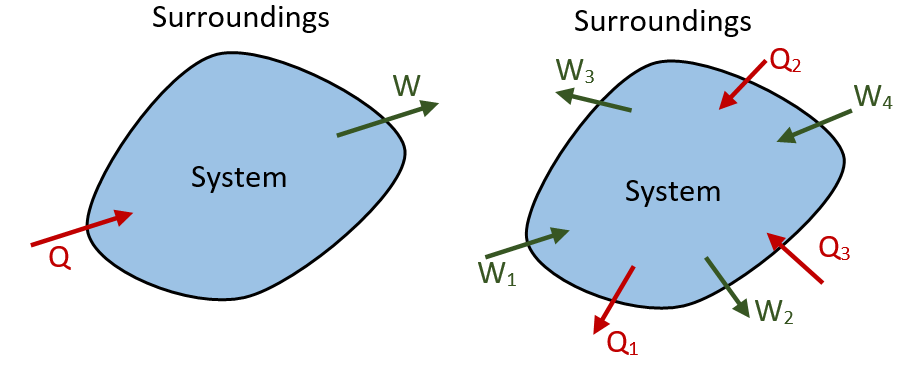### First Law for a Closed System Undergoing a Change of State or a Process

• Figure: Heat and Work interaction in a process• “If a system undergoes a change of state during which both heat transfer and work transfer are involved, the net energy transfer will be stored or accumulated within the system”.

• If Q is the amount of heat transferred to the system and W is the amount of work transferred from the system during the process as shown in figure and the net energy transfer (Q-W) will be stored in the system. Energy in storage is neither heat nor work and is given the name Internal Energy or simply the Energy of the system.
Q – W = ΔE  Or  Q = ΔE + W..........1
Where: ΔE is the increase in the energy of the system
Here: Q, W and ΔE are all expressed in the same units (Joule)

• Energy may be stored by a system in different modes. If there are more energy transfer quantities involved in the process as shown in figure. The first law gives-
[Q2 + Q3 – Q1] = [W2 + W3 –W1 – W4]..........2

• The first law is a particular formulation of the principle of the conservation of energy. Equation-1 can also be considered as the definition of ENERGY. This definition doesn’t give an absolute value of energy E, but only the change of energy ΔE for the process. It can however be shown that the energy has a definite value at every state of a system and is a property of the system.